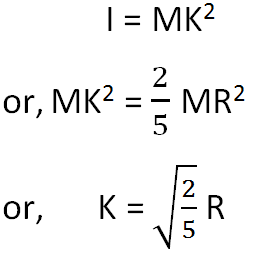Radius of gyration is defined as the distance from the axis of rotation to a point where the total mass of the body is supposed to be concentrated, so that the moment of inertia about the axis may remain the same. Simply, gyration is the distribution of the components of an object. It is denoted by K. In terms of radius of gyration, the moment of inertia of the body of mass M is given as,

`Inertia (I) = MK2`

Suppose a body consists of n particles each of mass m. Let r1, r2, r3, , rn be their perpendicular distances from the axis of rotation. Then, the moment of inertia I of the body about the axis of rotation is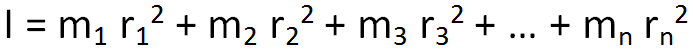If all the particles are of same mass m, then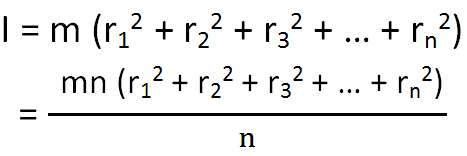Since mn = M, total mass of the body,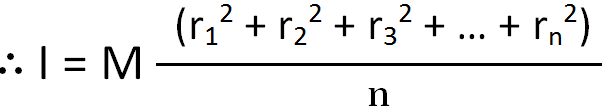From the above equations, we have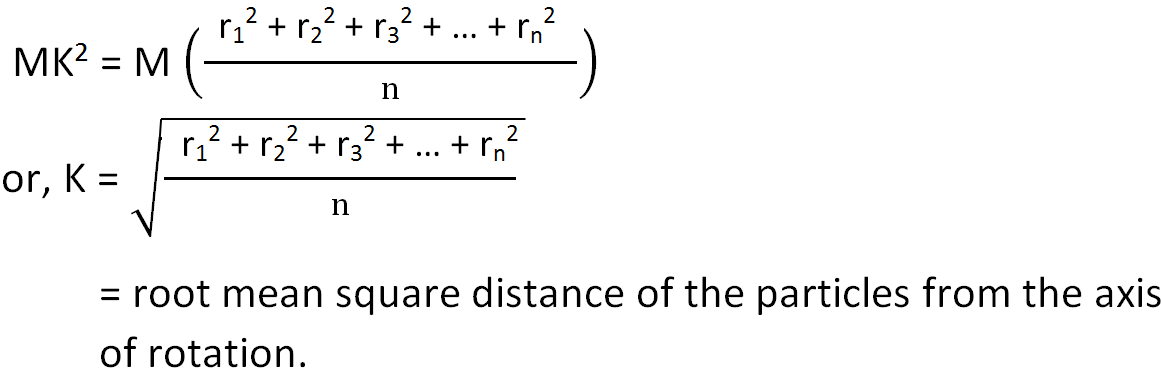Therefore, the radius of gyration of a body about a given axis may also be defined as the root mean square distance of the various particles of the body from the axis of rotation.

### Radius of Gyration of a Thin Rod

The moment of inertia of uniform thin rod of mass M and length l about an axis through its center and perpendicular to its length is given by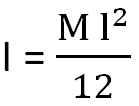If K is the radius of gyration of the rod about the axis, then we have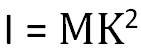From the above equations, we have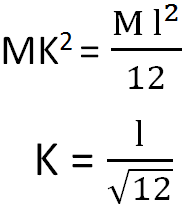### Radius of Gyration of a Solid Sphere

The moment of inertia for a solid sphere of radius R and mass M is given by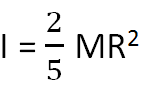If K is the radius of gyration of the solid sphere, then